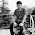### Stick Broken Into Three Pieces

Source: http://www.cut-the-knot.org/

Problem:
Assume a stick is broken at random into three pieces. What is the probability that the pieces can form a triangle?

Solve it in following cases:
1. Two break points are selected randomly (and distributed uniformly) on the stick.
2. The stick is first broken into two pieces. The longest (or rather, not the shortest) is then broken into two.
3. The stick is first broken into two pieces. A piece randomly selected with probability 1/2 is then broken into two.
4. The stick is first broken into two pieces. A piece randomly selected with the probability proportional to its length is then broken into two.
Update (Dec 22, 2010)
Solution posted by me in comments!

1.2.